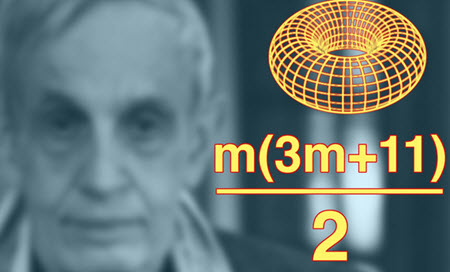# Nash embedding theoremThe Nash embeddings theorems state that every Reimannian manifold can be isometrically embedded into some Euclidean space. Isometrically embedded = embedded in a way that preserves the length of every path. Bending is premitted, but not stretching, tearing, etc. Curves drawn on the surface must retain the same arclenght even after bending.

The Nash embeddings theorems are named after the matematician John Nash, who published his C1-theorem in 1954 and his Ck-theorem in 1956. The real analytic theorem was first treated by Nash in 1966.

## What is a Reimannian manifold?

In differential geometry, a (smooth) Riemannian manifold (M, g) is a real, smooth manifold M equipped with an inner product gp on the tangent space TpM at each point p that varies smoothly from point to point in the sense that if X and Y are differentiable vector fields on M, then pgp(X|p, Y|p) is a smooth function.

The Riemannian manifold is named after the German mathematician Bernhard Riemann (1826 – 1866).

## The C1-theorem

This theorem is for continuously differentiable (C1) embeddings.

Unlike the Ck-theorem, this one has a very simple proof and leads to some very counter-intuitive conclusions.

Nash’s original theorem had the condition n ≥ m+2 instead of n ≥ m+1, but the theorem was later generalized by Nicolaas Kuiper.

### Nash–Kuiper theorem (C1 embedding theorem)

Let (M,g) be a Riemannian manifold and ƒ: MmRn a short C∞-embedding (or immersion) into Euclidean space Rn, where nm+1. Then for arbitrary ε > 0 there is an embedding (or immersion) ƒε: MmRn which is

1. in class C1,
2. isometric: for any two vectors v,w ∈ Tx(M) in the tangent space at xM,

g ( v , w ) = ⟨ d f ε ( v ) , d f ε ( w ) ⟩

3. ε-close to ƒ:

| f ( x ) − f ε ( x ) | < ϵ   ∀   x ∈ M

## The Ck-theorem

This theorem is for analytic embeddings or embeddings that are smooth of class Ck, 3 ≤ k ≤ ∞.

This theorem has a very technical and complex proof, but unlike the C1-theorem, it doesn’t lead to counter-intuitive conclusions.

The technical statement found in Nash’s paper from 1956 looks like this:

 If M is a given m-dimensional Riemannian manifold (analytic or of class Ck, 3 ≤ k ≤ ∞), then there exists a number n (with n ≤ m(3m+11)/2 if M is a compact manifold, or n ≤ m(m+1)(3m+11)/2 if M is a non-compact manifold) and an injective map ƒ: M → Rn (also analytic or of class Ck) such that for every point p of M, the derivative dƒp is a linear map from the tangent space TpM to Rn which is compatible with the given inner product on TpM and the standard dot product of Rn in the following sense: ⟨ u , v ⟩ = d f p ( u ) ⋅ d f p ( v ) for all vectors u, v in TpM.